The other day I encountered a SAS Knowledge Base article that shows how to count the number of missing and nonmissing values for each variable in a data set. However, the code is a complicated macro that is difficult for a beginning SAS programmer to understand. (Well, it was hard for me to understand!) The code not only counts the number of missing values for each variable, but also creates a SAS data set with the complete results. That's a nice bonus feature, but it contributes to the complexity of the macro.

This article simplifies the process and shows an alternative way to count the number of missing and nonmissing values for each variable in a data set.

### The easy case: Count missing values for numeric variables

If you are only interested in the number of missing values for numeric variables, then a single call to the MEANS procedure computes the answer:

```/* create sample data */ data one; input a \$ b \$ c \$ d e; cards; a . a 1 3 . b . 2 4 a a a . 5 . . b 3 5 a a a . 6 a a a . 7 a a a 2 8 ; run;   proc means data=one NMISS N; run;```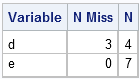In many SAS procedures, including PROC MEANS, you can omit the VAR statement in order to operate on all relevant variables. For the MEANS procedure, "relevant" means "numeric."

### Count missing values for all variables

The MEANS procedure computes statistics for numeric variables, but other SAS procedures enable you to count the number of missing values for character and numeric variables.

The FREQ procedure is a SAS workhorse that I use almost every day. To get the FREQ procedure to count missing values, use three tricks:

1. Specify a format for the variables so that the missing values all have one value and the nonmissing values have another value. PROC FREQ groups a variable's values according to the formatted values.
2. Specify the MISSING and MISSPRINT options on the TABLES statement.
3. Use the _CHAR_ and _NUM_ keywords on the TABLES statement to specify that the FREQ procedure should compute statistics for all character or all numeric variables.

The following statements count the number of missing and nonmissing values for every variable: first the character variables and then the numeric ones.

```/* create a format to group missing and nonmissing */ proc format; value \$missfmt ' '='Missing' other='Not Missing'; value missfmt . ='Missing' other='Not Missing'; run;   proc freq data=one; format _CHAR_ \$missfmt.; /* apply format for the duration of this PROC */ tables _CHAR_ / missing missprint nocum nopercent; format _NUMERIC_ missfmt.; tables _NUMERIC_ / missing missprint nocum nopercent; run;```### Using the SAS/IML language to count missing values

In the SAS/IML Language, you can use the COUNTN and COUNTMISS functions that were introduced in SAS/IML 9.22. Strictly speaking, you need to use only one of the functions, since the result of the other is determined by knowing the number of observations in the data set. For the sake of the example, I'll be inefficient and use both of the functions.

As is the case for the PROC FREQ example, the trick is to use the _CHAR_ and _NUM_ keywords to read in and operate on the character and numeric variables in separate steps:

```proc iml; use one; read all var _NUM_ into x[colname=nNames]; n = countn(x,"col"); nmiss = countmiss(x,"col");   read all var _CHAR_ into x[colname=cNames]; close one; c = countn(x,"col"); cmiss = countmiss(x,"col");   /* combine results for num and char into a single table */ Names = cNames || nNames; rNames = {" Missing", "Not Missing"}; cnt = (cmiss // c) || (nmiss // n); print cnt[r=rNames c=Names label=""];```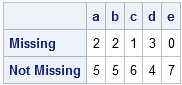This is similar to the output produced by the macro in the SAS Knowledge Base article. You can also write the cnt matrix to a data set, if necessary.Looping is essential to statistical programming. Whether you need to iterate over parameters in an algorithm or indices in an array, a loop is often one of the first programming constructs that a beginning programmer learns.

Today is the first anniversary of this blog, which is named The DO Loop, so it seems appropriate to blog about DO loops in SAS. I'll describe looping in the SAS DATA step and compare it with looping in the SAS/IML language.

### Loops in SAS

Loops are fundamental to programming because they enable you to repeat a computation for various values of parameters. Different languages use different keywords to define the iteration statement. The most well-known statement is the "for loop," which is used by C/C++, MATLAB, R, and other languages. Older languages, such as FORTRAN and SAS, call the iteration statement a "do loop," but it is exactly the same concept.

### DO loops in the DATA step

The basic iterative DO statement in SAS has the syntax DO value = start TO stop. An END statement marks the end of the loop, as shown in the following example:

```data A; do i = 1 to 5; y = i**2; /* values are 1, 4, 9, 16, 25 */ output; end; run;```

By default, each iteration of a DO statement increments the value of the counter by 1, but you can use the BY option to increment the counter by other amounts, including non-integer amounts. For example, each iteration of the following DATA step increments the value i by 0.5:

```data A; do i = 1 to 5 by 0.5; y = i**2; /* values are 1, 2.25, 4, 6.25, ..., 25 */ output; end; run;```

You can also iterate "backwards" by using a negative value for the BY option: do i=5 to 1 by -0.5.

### DO loops in SAS/IML Software

A basic iterative DO statement in the SAS/IML language has exactly the same syntax as in the DATA step, as shown in the following PROC IML statements:

```proc iml; x = 1:4; /* vector of values {1 2 3 4} */ do i = 1 to 5; z = sum(x##i); /* 10, 30, 100, 354, 1300 */ end;```

In the body of the loop, z is the sum of powers of the elements of x. During the ith iteration, the elements of x are raised to the ith power. As mentioned in the previous section, you can also use the BY option to increment the counter by non-unit values and by negative values.

### Variations on the DO loop: DO WHILE and DO UNTIL

On occasion, you might want to stop iterating if a certain condition occurs. There are two ways to do this: you can use the WHILE clause to iterate as long as a certain condition holds, or you can use the UNTIL clause to iterate until a certain condition holds.

You can use the DO statement with a WHILE clause to iterate while a condition is true. The condition is checked before each iteration, which implies that you should intialize the stopping condition prior to the loop. The following statements extend the DATA step example and iterate as long as the value of y is less than 20:

```data A; y = 0; do i = 1 to 5 by 0.5 while(y < 20); y = i**2; /* values are 1, 2.25, 4, 6.25, ..., 16 */ output; end; run;```

You can use the iterative DO statement with an UNTIL clause to iterate until a condition becomes true. The UNTIL condition is evaluated at the end of the loop, so you do not have to initialize the condition prior to the loop. The following statements extend the PROC IML example. The iteration stops after the value of z exceeds 200.

```proc iml; x = 1:4; do i = 1 to 5 until(z > 200); z = sum(x##i); /* 10, 30, 100, 354 */ end;```

In these examples, the iteration stopped because the WHILE or UNTIL condition was satisfied. If the condition is not satisfied when i=5 (the last value for the counter), the loop stops anyway. Consequently, the examples have two stopping conditions: a maximum number of iterations and the WHILE or UNTIL criterion. SAS also supports a DO WHILE and DO UNTIL syntax that does not involve using a counter variable.

### Looping over a set of items (foreach)

Some languages support a "foreach loop" that iterates over objects in a collection. SAS doesn't support that syntax directly, but there is a variant of the DO loop in which you can iterate over values in a specified list. The syntax in the DATA step is to specify a list of values (numeric or character) after the equal sign. The following example iterates over a few terms in the Fibonacci sequence:

```data A; do v = 1, 1, 2, 3, 5, 8, 13, 21; y = v/lag(v); output; end; run;```

The ratio of adjacent values in a Fibonacci sequence converges to the golden ratio, which is 1.61803399....

The SAS/IML language does not support this syntax, but does enable you to iterate over values that are contained in a vector (or matrix). The following statements create a vector, v, that contains the Fibonacci numbers. An ordinary DO loop is used to iterate over the elements of the vector. At the end of the loop, the vector z contains the same values as the variable Y that was computed in the DATA step.

```proc iml; v = {1, 1, 2, 3, 5, 8, 13, 21}; z = j(nrow(v),1,.); /* initialize ratio to missing values */ do i = 2 to nrow(v); z[i] = v[i]/v[i-1]; end;```

### Avoid unnecessary loops in the SAS/IML Language

I have some advice on using DO loops in SAS/IML language: look carefully to determine if you really need a loop. The SAS/IML language is a matrix/vector language, so statements that operate on a few long vectors run much faster than equivalent statements that involve many scalar quantities. Experienced SAS/IML programmers rarely operate on each element of a vector. Rather, they manipulate the vector as a single quantity. For example, the previous SAS/IML loop can be eliminated:

```proc iml; v = {1, 1, 2, 3, 5, 8, 13, 21}; idx = 2:nrow(v); z = v[idx]/v[idx-1];```

This computation, which computes the nonmissing ratios, is more efficient than looping over elements. For other tips and techniques that make your SAS/IML programs more efficient, see my book Statistical Programming with SAS/IML Software.I previously showed how to generate random numbers in SAS by using the RAND function in the DATA step or by using the RANDGEN subroutine in SAS/IML software. These functions generate a stream of random numbers. (In statistics, the random numbers are usually a sample from a distribution such as the uniform or the normal distribution.) You can control the stream by setting the seed for the random numbers. The random number seed is set by using the STREAMINIT subroutine in the DATA step or the RANDSEED subroutine in the SAS/IML language.

A random number seed enables you to generate the same set of random numbers every time that you run the program. This seems like an oxymoron: if they are the same every time, then how can they be random? The resolution to this paradox is that the numbers that we call "random" should more accurately be called "pseudorandom numbers." Pseudorandom numbers are generated by an algorithm, but have statistical properties of randomness. A good algorithm generates pseudorandom numbers that are indistinguishable from truly random numbers. The random number generator used in SAS is the Mersenne-Twister random number generator (Matsumoto and Nishimura, 1998), which is known to have excellent statistical properties.

Why would you want a reproducible sequence of random numbers? Documentation and testing are two important reasons. When I write SAS code and publish it on this blog, in a book, or in SAS documentation, it is important that SAS customers be able to run the code and obtain the same results.

### Random number streams in the DATA step

The STREAMINIT subroutine is used to set the random number seed for the RAND function in the DATA step. The seed value controls the sequence of random numbers. Syntactically, you should call the STREAMINIT subroutine one time per DATA step, prior to the first invocation of the RAND function. This ensures that when you run the DATA step later, it produces the same pseudorandom numbers.

If you start a new DATA step, you can specify a new seed value. If you use a seed value of 0, or if you do not specify a seed value, then the system time is used to determine the seed value. In this case, the random number stream is not reproducible.

To see how random number streams work, each of the following DATA step creates five random observations. The first and third data sets use the same random number seed (123), so the random numbers are identical. The second and fourth variables both use the system time (at the time that the RAND function is first called) to set the seed. Consequently, those random number streams are different. The last data set contains random numbers generated by a different seed (456). This stream of numbers is different from the other streams.

```data A(drop=i); call streaminit(123); do i = 1 to 5; x123 = rand("Uniform"); output; end; run; data B(drop=i); call streaminit(0); do i = 1 to 5; x0 = rand("Uniform"); output; end; run; data C(drop=i); call streaminit(123); do i = 1 to 5; x123_2 = rand("Uniform"); output; end; run; data D(drop=i); /* no call to streaminit */ do i = 1 to 5; x0_2 = rand("Uniform"); output; end; run; data E(drop=i); call streaminit(456); do i = 1 to 5; x456 = rand("Uniform"); output; end; run; data AllRand; merge A B C D E; run; /* concatenate */ proc print data=AllRand; run;```Notice that the STREAMINIT subroutine, if called, is called exactly one time at the beginning of the DATA step. It does not make sense to call STREAMINIT multiple times within the same DATA step; subsequent calls are ignored. In the one DATA step (D) that does not call STREAMINIT, the first call to the RAND function implicitly calls STREAMINIT with 0 as an argument.

If a single program contains multiple DATA steps that generate random numbers (as above), use a different seed in each DATA step or else the streams will not be independent. This is also important if you are writing a macro function that generates random numbers. Do not hard-code a seed value. Rather, enable the user to specify the seed value in the syntax of the function.

### Random number streams in PROC IML

So that it is easier to compare random numbers generated in SAS/IML with random numbers generated by the SAS DATA step, I display the table of SAS/IML results first: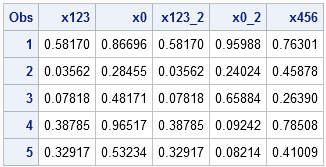These numbers are generated by the RANDGEN and RANDSEED subroutines in PROC IML. The numbers are generated by five procedure calls, and the random number seeds are identical to those used in the DATA step example. The first and third variables were generated from the seed value 123, the second and fourth variables were generated by using the system time, and the last variable was generated by using the seed 456. The following program generates the data sets, which are then concatenated together.

```proc iml; call randseed(123); x = j(5,1); call randgen(x, "Uniform"); create A from x[colname="x123"]; append from x; proc iml; call randseed(0); x = j(5,1); call randgen(x, "Uniform"); create B from x[colname="x0"]; append from x; proc iml; call randseed(123); x = J(5,1); call randgen(x, "Uniform"); create C from x[colname="x123_2"]; append from x; proc iml; /* no call to randseed */ x = J(5,1); call randgen(x, "Uniform"); create D from x[colname="x0_2"]; append from x; proc iml; call randseed(456); x = J(5,1); call randgen(x, "Uniform"); create E from x[colname="x456"]; append from x; quit; data AllRandgen; merge A B C D E; run; proc print data=AllRandgen; run;```

Notice that the numbers in the two tables are identical for columns 1, 3, and 5. The DATA step and PROC IML use the same algorithm to generate random numbers, so they produce the same stream of random values when given the same seed.

### Summary

• To generate random numbers, use the RAND function (for the DATA step) and the RANDGEN call (for PROC IML).
• To create a reproducible stream of random numbers, call the STREAMINIT (for the DATA step) or the RANDSEED (for PROC IML) subroutine prior to calling RAND or RANDGEN. Pass a positive value (called the seed) to the routines.
• To initialize a stream of random numbers that is not reproducible, call STREAMINIT or RANDSEED with the seed value 0.
• To ensure independent streams within a single program, use a different seed value in each DATA step or procedure.One of the highly visible changes in SAS 9.3 is the fact that the old LISTING destination is no longer the default destination for ODS output. Instead, the HTML destination is the default.

One positive consequence of this is that ODS graphics and tables are interlaced in the output. Another is that complicated tables are easier to read because SAS can use styles (colors, fonts, and cell shading) to make tables more readable. For example, the row and column headings of a table might be a different color than the cells of the table.

However, if you are like me, you occasionally like to clear the output window so that it doesn't get too crowded. In SAS 9.2, clearing the Output Window (by which I mean the LISTING destination) in the SAS Windowing Environment was easy: select Edit > Clear All or select the Output Window and click the New icon (or File > New).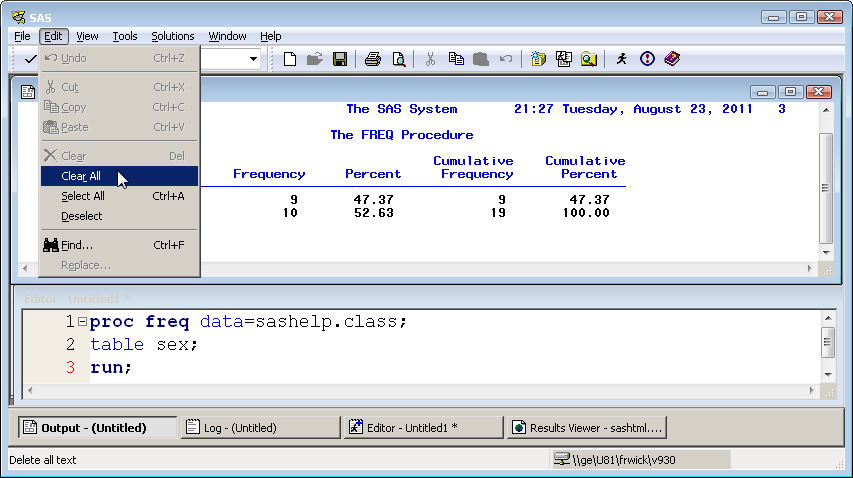I assumed that I could clear the HTML Results Viewer in SAS 9.3 the same way, but as the following image shows, the Edit > Clear All menu item is disabled!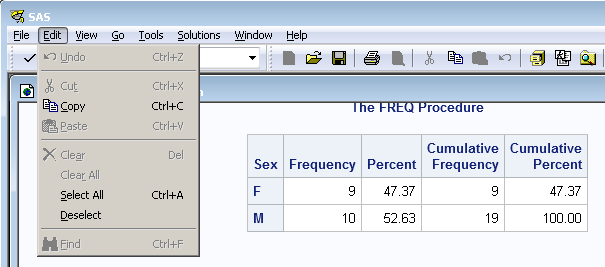No worries, though. You can clear the Results Viewer programmatically by submitting the following statements:

```ods html close; /* close previous */ ods html; /* open new */```

The Results Viewer will not immediately look empty, but the next time that you generate output you will see that the Results Viewer no longer includes the older content.

There is a SAS Usage Note that describes other ways to clear the contents of the Results Viewer. Thanks to the fine folks on the SAS-L Mailing List for asking this question and for linking to the SAS Knowledge Base article.Exploring correlation between variables is an important part of exploratory data analysis. Before you start to model data, it is a good idea to visualize how variables related to one another. Zach Mayer, on his Modern Toolmaking blog, posted code that shows how to display and visualize correlations in R. This is such a useful task that I want to repeat it in SAS software.

### Basic correlations and a scatter plot matrix

Mayer's used Fisher's iris data for his example, so I will, too. The following statement uses the CORR procedure to compute the correlation matrix and display a scatter plot matrix for the numerical variables in the data:

```proc corr data=sashelp.iris plots=matrix(histogram); run;```Notice that by omitting the VAR statement, the CORR procedure analyzes all numerical variables in the data set.

The PLOTS=MATRIX option displays a scatter plot matrix. In SAS 9.3, ODS graphics are turned on by default. (In SAS 9.2, you need to submit ODS graphics on; prior to the PROC CORR statement.) The result is a "quick-and-dirty" visualization of pairwise relationships and the distribution of each variable (along the diagonal). This is the beauty of ODS graphics: the procedures automatically create graphs that are appropriate for an analysis.

### A fancier a scatter plot matrix

Mayer also showed some fancier graphs. You can use the SGSCATTER procedure to re-plot the data, but with observations colored according to values of the Species variable, and with a kernel density estimate overlaid on the histogram.

```proc sgscatter data=sashelp.iris; matrix SepalLength--PetalLength /group=Species diagonal=(histogram kernel); run;```Notice how I specified the variables. Did you know that you can specify a range of consecutive variables by using a double-dash? This SAS syntax isn't widely known, but can be very useful.

### More options, more details

I don't usually add smoothers to my scatter plot matrices because I think it gives the false impression that certain variables are response variables. I prefer to focus on correlation first and save modeling for later in the analysis. However, Mayer showed some loess smoothers on his plots, so I feel obligated to show SAS users how to produce similar displays.

The observant reader will have noticed that there are no scales or tick marks on the scatter plot matrices that I've shown so far. The reason is that axes and scales can distract from the primary goal of the exploratory analysis, which is to give an overview of the data and to see potential pairwise relationships. In Tufte's jargon, the scatter plot matrices that I've shown have a large data-to-ink ratio (Ch. 4, The Visual Display of Quantitative Information).

However, scatter plot matrices also can serve another purpose. During the modeling phase of data analysis they can serve as small multiples that enable you to quickly compare and contrast a sequence of related displays. In this context, scales, tick marks, and statistical smoothers are more relevant.

In general, you can use the SGPANEL procedure to display small multiples. However, I'll use the SGSCATTER procedure again to show how you can add more details to the display. Instead of using the MATRIX statement, I will use the PLOT statement to control exactly with pairs of variables I want to plot. If I think that PetalWidth variable explains the other variables, I can use the LOESS option to add a loess smoother to the scatter plots, as shown in the following example:

```proc sgscatter data=sashelp.iris; plot (SepalLength SepalWidth PetalLength)*PetalWidth / group=Species loess rows=1 grid; run;```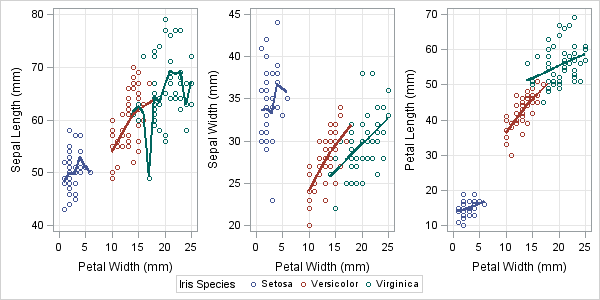Notice that the loess smoothers are added for each group because the GROUP= option is specified. If, instead, you want to smooth the data regardless of the group variable, you can specify the LOESS=(NOGROUP) option, which produces smoothers similar to those shown by Mayer.In SAS, you can generate a set of random numbers that are uniformly distributed by using the RAND function in the DATA step or by using the RANDGEN subroutine in SAS/IML software. (These same functions also generate samples from other common distributions such as binomial and normal.) The syntax is simple. The following DATA step creates a data set that contains 10 random uniform numbers in the range [0,1]:

```data A; call streaminit(123); /* set random number seed */ do i = 1 to 10; u = rand("Uniform"); /* u ~ U[0,1] */ output; end; run;```

The syntax for the SAS/IML program is similar, except that you can avoid the loop (vectorize) by allocating a vector and then filling all elements by using a single call to RANDGEN:

```proc iml; call ranseed(123); /* set random number seed */ u = j(10,1); /* allocate */ call randgen(u, "Uniform"); /* u ~ U[0,1] */```

### Random uniform on the interval [a,b]

If you want generate random numbers on the interval [a,b], you have to scale and translate the values that are produced by RAND and RANDGEN. The width of the interval [a,b] is b-a, so the following statements produce random values in the interval [a,b]:

``` a = -1; b = 1; /* example values */ x = a + (b-a)*u;```

The same expression is valid in the DATA step and the SAS/IML language.

### Random integers

You can use the FLOOR or CEIL functions to transform (continuous) random values into (discrete) random integers. In statistical programming, it is common to generate random integers in the range 1 to Max for some value of Max, because you can use those values as observation numbers (indices) to sample from data. The following statements generate random integers in the range 1 to 10:

``` Max = 10; k = ceil( Max*u ); /* uniform integer in 1..Max */```

If you want random integers between 0 and Max or between Min and Max, the FLOOR function is more convenient:

``` Min = 5; n = floor( (1+Max)*u ); /* uniform integer in 0..Max */ m = min + floor( (1+Max-Min)*u ); /* uniform integer in Min..Max */```

Again, the same expressions are valid in the DATA step and the SAS/IML language.

### Putting it all together

The following DATA step demonstrates all the ideas in this blog post and generates 1,000 random uniform values with various properties:

```%let NObs = 1000; data Unif(keep=u x k n m); call streaminit(123); a = -1; b = 1; Min = 5; Max = 10; do i = 1 to &NObs; u = rand("Uniform"); /* U[0,1] */ x = a + (b-a)*u; /* U[a,b] */ k = ceil( Max*u ); /* uniform integer in 1..Max */ n = floor( (1+Max)*u ); /* uniform integer in 0..Max */ m = min + floor((1+Max-Min)*u); /* uniform integer in Min..Max */ output; end; run;```

You can use the UNIVARIATE and FREQ procedures to see how closely the statistics of the sample match the characteristics of the populations. The PROC UNIVARIATE output is not shown, but the histograms show that the sample data for the u and x variables are, indeed, uniformly distributed on [0,1] and [-1,1], respectively. The PROC FREQ output shows that the k, n, and m variables contain integers that are uniformly distributed within their respective ranges. Only the output for the m variable is shown.

```proc univariate data=Unif; var u x; histogram u/ endpoints=0 to 1 by 0.05; histogram x/ endpoints=-1 to 1 by 0.1; run;   proc freq data=Unif; tables k n m / chisq; run;```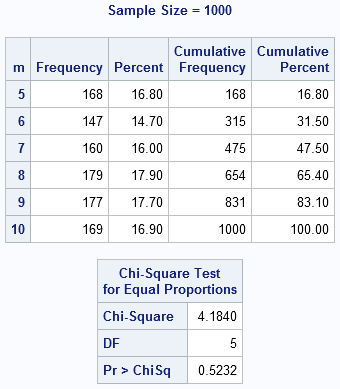One of the great innovations with SAS 9.3 is the focus on ODS statistical graphics.

"Wait a minute," you're thinking, "weren't ODS graphics added in SAS 9.2?"

Yes, that's true.  But with SAS 9.3 there is even more capability: more analytical SAS procedures support the graphs, and there are more features in the specialized graph procedures such as SGPLOT.

But even more remarkable is the change in SAS display manager to bring ODS graphics into focus.  Now HTML output (not text listing) is the default output from SAS display manager.  And a new ODS style, named "HTMLBlue", has been designed to show the graphs in a crisp and colorful way.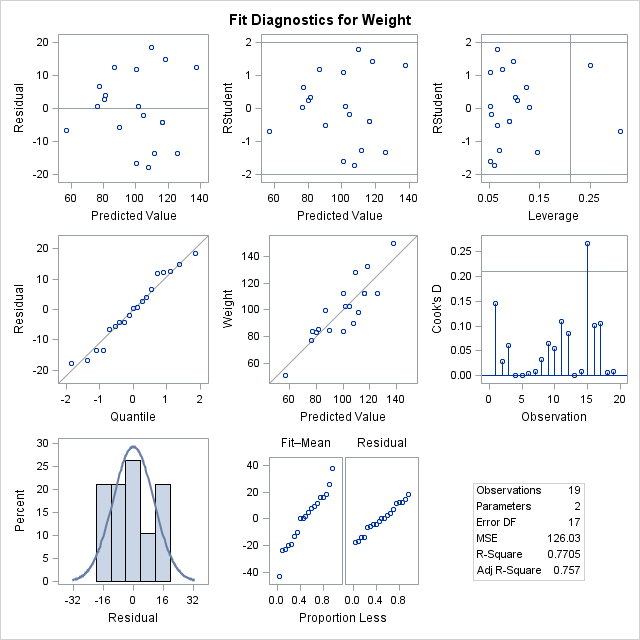If you are using SAS Enterprise Guide 4.3, you don't have HTMLBlue in your list of available styles.  But you can add it with a few simple steps.

### 1. Use SAS to create a copy of the HTMLBlue stylesheet.

```filename out temp; /* change the location of the css fileref as necessary */ filename css "c:\temp\htmlblue.css"; ods html file=out style=htmlblue stylesheet=css; proc print data=sashelp.class; run; ods html close;```

You can run this code in a SAS window or in SAS Enterprise Guide, making sure that you are able to write to the location that the CSS fileref is mapped to.  After running the program, the htmlblue.css file will appear in the location specified in the program.

### 2. Copy the htmlblue.css file to the Styles subfolder in your SAS Enterprise Guide application directory.

Depending on how you installed SAS Enterprise Guide, that location might be:

C:\Program Files\SAS\EnterpriseGuide\4.3\Styles
or
C:\Program Files\SASHome\x86\SASEnterpriseGuide\4.3\Styles

### 3. In SAS Enterprise Guide, change the preferences for your results output to use the new HTMLBlue style.

The style will automatically appear in the list of available styles.  For example, to change the default output for SAS Report, select Tools->Options->Results->SAS Report, and select "htmlblue" from the list of styles.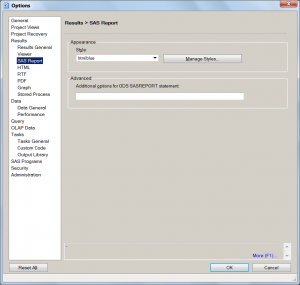And even though the style is named "HTMLBlue", you don't have to use it for just HTML.  It's just as attractive when using SAS Report.  Of course you can also use it for RTF and PDF output as well, although you might prefer a cleaner style such as Journal for printed output.  The SAS OnlineDoc for statistical graphics contains a comparison of the various styles, with considerable focus on the details for the graphics.It seems like such a simple problem: how can you reliably compute the age of someone or something? Susan lamented the subtle issues using the YRDIF function exactly 1.0356164384 years ago.

Sure, you could write your own function for calculating such things, as I suggested 0.1753424658 years ago.

Or you could ask your colleagues in the discussion forums, as I noticed some people doing just about 3.7890410959 years ago.

Or, now that SAS 9.3 is available, you can take advantage of the new AGE basis that the YRDIF function supports. For example:

data _null_;
x = yrdif('29JUN2010'd,today(),'AGE');
y = yrdif('09MAY2011'd,today(),'AGE');
z = yrdif('27SEP2007'd,today(),'AGE');
put x= y= z=;
run;

Yields:

x=1.0356164384 y=0.1753424658 z=3.7890410959

This new feature and many more were added in direct response to customer feedback from Susan and others. And that's a practice that never gets old.It seems like such a simple problem: how can you reliably compute the age of someone or something? Susan lamented the subtle issues using the YRDIF function exactly 1.0356164384 years ago.

Sure, you could write your own function for calculating such things, as I suggested 0.1753424658 years ago.

Or you could ask your colleagues in the discussion forums, as I noticed some people doing just about 3.7890410959 years ago.

Or, now that SAS 9.3 is available, you can take advantage of the new AGE basis that the YRDIF function supports. For example:

```data _null_; x = yrdif('29JUN2010'd,today(),'AGE'); y = yrdif('09MAY2011'd,today(),'AGE'); z = yrdif('27SEP2007'd,today(),'AGE'); put x= y= z=; run;```

Yields:

```x=1.0356164384 y=0.1753424658 z=3.7890410959
```

This new feature and many more were added in direct response to customer feedback from Susan and others. And that's a practice that never gets old.SAS Enterprise Guide sets values for several useful SAS macro variables when it connects to a SAS session, including one macro variable, &_CLIENTPROJECTPATH, that contains the name and path of the current SAS Enterprise Guide project file.

(To learn about this and other macro variables that SAS Enterprise Guide assigns, look in Help->SAS Enterprise Guide help, and search the Index for "macro variables".)

If your SAS program knows the path of your project file, then you can use that information to make your project more "portable", and reference data resources using paths that are relative to the project location, instead of having to hard-code absolute paths into your program.

For example, I've been working with some data from DonorsChoose.org, and I've captured that work in a project file. After saving the project, I can easily get the name of the project file by checking the value of &_CLIENTPROJECTPATH:

15    %put &_clientprojectpath;
'C:\DataSources\DonorsChoose\DonorsChoose.egp'

If you can get the full path of the project file, then you can build that information into a SAS program so that as you move the project file and its "assets" (such as data), you don't need to make changes to the project to accommodate a new project folder "home". Here is the bit of code that takes the project file location and distills a path from it, and then uses it to assign a path-based SAS library.

/* a bit of code to detect the local project path                          */
/* NOTE: &_CLIENTPROJECTPATH is set only if you saved the project already! */
%let localProjectPath =
%sysfunc(substr(%sysfunc(dequote(&_CLIENTPROJECTPATH)), 1,
%sysfunc(findc(%sysfunc(dequote(&_CLIENTPROJECTPATH)), %str(/\), -255 ))));

libname DC "&localProjectPath";

Here's the output:

21  libname DC "&localProjectPath";
NOTE: Libref DC was successfully assigned as follows:
Engine:        V9
Physical Name: C:\DataSources\DonorsChoose

This allows me to keep the project together with the data that it consumes and creates. And I can easily share the project and data with a colleague, who can store it in a different folder on his/her machine, and it should work just the same without any changes.